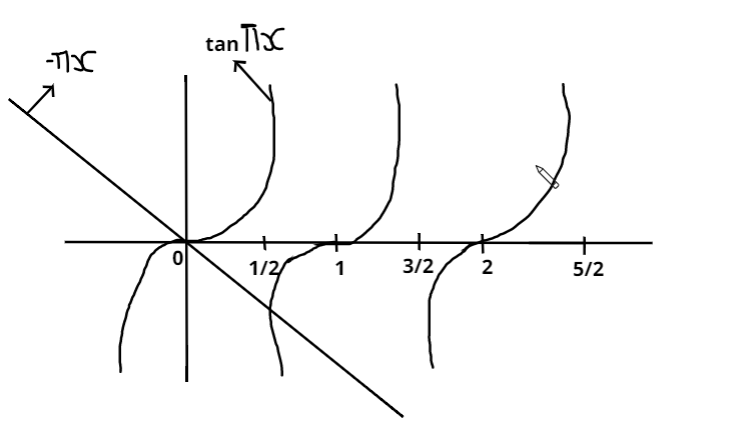QuestionAnswers

# Let$f\left( x \right) = x\sin \pi x,{\text{ }}x > 0$ . Then for all natural numbers $n,{\text{ }}f'\left( x \right)$ vanishes at:(A). A unique point in the interval $\left( {n,n + \dfrac{1}{2}} \right)$ (B). A unique point in the interval $\left( {n + \dfrac{1}{2},n + 1} \right)$ (C). A unique point in the interval$\left( {n,n + 1} \right)$ (D). Two points in the interval $\left( {n,n + 1} \right)$Hint- To solve this question first we have to find the differentiation of the given function, then equate this to zero. and we get the required answer.

Complete step-by-step solution -

Now given that,
$f\left( x \right) = x\sin \pi x,{\text{ }}x > 0$
And we have to find $f'\left( x \right)$
Now to differentiate it we will apply product rule.
According to product rule,
$\dfrac{{d\left( {uv} \right)}}{{dx}} = u\dfrac{{dv}}{{dx}} + v\dfrac{{du}}{{dx}}$
Now,
$\dfrac{{df\left( x \right)}}{{dx}} = f'\left( x \right) = \sin \left( {\pi x} \right) \cdot \dfrac{{dx}}{{dx}} + x\dfrac{{d\sin \left( {\pi x} \right)}}{{dx}} \\ {\text{or }}f'\left( x \right) = \sin \left( {\pi x} \right) + x\dfrac{{d\sin \left( {\pi x} \right)}}{{d\left( {\pi x} \right)}} \times \dfrac{{d\left( {\pi x} \right)}}{{dx}}{\text{ }}\left( {{\text{By chain rule}}} \right){\text{ }} \\ {\text{or }}f'\left( x \right) = \sin \left( {\pi x} \right) + x\cos \left( {\pi x} \right) \cdot \pi \\ {\text{or }}f'\left( x \right) = \sin \left( {\pi x} \right) + \cos \pi x\left( {\pi x} \right) \\$
Now we have to find $f'\left( x \right)$ vanishes at, for that we will put the value of $f'\left( x \right) = 0$
$f'\left( x \right) = \sin \left( {\pi x} \right) + \cos \pi x\left( {\pi x} \right) = 0 \\ {\text{or - }}\left[ {\cos \left( {\pi x} \right)} \right]\pi x = \sin \left( {\pi x} \right) \\ {\text{or }}\dfrac{{\sin \left( {\pi x} \right)}}{{\cos \left( {\pi x} \right)}} = - \pi x \\ {\text{or }}\tan \left( {\pi x} \right) = - \pi x \\$
Now let’s draw the graph of $\tan \left( {\pi x} \right) = - \pi x$Now from the graph it is pretty much clear that $- \pi x$line is cutting $\tan \pi x$ for every $n + \dfrac{1}{2}$ but $n$ belongs to natural number, so it would be cutting a unique point between $\left( {n + \dfrac{1}{2},n + 1} \right)$ because as we see that it first cuts between $\dfrac{1}{2}$ to $1$ then it again cuts somewhere at $\dfrac{3}{2}$ and so on. Therefore there is a gap of $1$
$\Rightarrow \pi x \in \left( {\dfrac{{2n + 1}}{2}\pi ,\left( {n + 1} \right)\pi } \right) \\ \Rightarrow x \in \left( {n + \dfrac{1}{2},n + 1} \right){\text{ and also }}x \in \left( {n,n + 1} \right). \\$
Therefore, the correct option will be (B) and (C).
Note- These types of questions can be solved by using the concept of maxima and minima. In this question we simply find the value of $f'\left( x \right)$ and then we observe it in the graph and we get our answer.

Electromagnetic Spectrum X-raysSigma Bond and Pi BondTan 0 DegreesCBSE Class 12 Maths FormulasDifference Between Left and Right VentricleRight Angled Triangle ConstructionsCBSE Class 12 Maths Chapter-12 Linear Programming FormulaDifference Between Left Kidney and Right KidneyCBSE Class 6 Maths Chapter 12 - Ratio and Proportion FormulasImportant Questions with Answers for CBSE Class 6 to 12 - All SubjectsImportant Questions for CBSE Class 12 Maths Chapter 12 - Linear ProgrammingImportant Questions for CBSE Class 8 Maths Chapter 12 - Exponents and PowersImportant Questions for CBSE Class 12 Maths Chapter 1 - Relations and FunctionsNCERT Books for Class 6 to 12 - All SubjectsImportant Questions for CBSE Class 12 Maths Chapter 5 - Continuity and DifferentiabilityImportant Questions for CBSE Class 12 Maths Chapter 7 - IntegralsImportant Questions for CBSE Class 12 Maths Chapter 13 - ProbabilityImportant Questions for CBSE Class 6 Maths Chapter 12 - Ratio and ProportionImportant Questions for CBSE Class 12 Maths Chapter 4 - DeterminantsCBSE Class 12 Maths Question Paper 2020Maths Question Paper for CBSE Class 12 - 2013Previous Year Question Paper for CBSE Class 12 Maths - 2014Maths Question Paper for CBSE Class 12 - 2016 Set 1 CMaths Question Paper for CBSE Class 12 - 2016 Set 1 EMaths Question Paper for CBSE Class 12 - 2016 Set 1 SHindi Question Paper for CBSE Class 12Maths Question Paper for CBSE Class 12 - 2016 Set 1 NChemistry Question Paper for CBSE Class 12English Question Paper for CBSE Class 12NCERT Solutions for Class 12 Maths ExercisesRD Sharma Class 12 Maths Solutions Chapter 18 - Maxima and MinimaRD Sharma Class 12 Solutions Chapter 18 - Maxima and Minima (Ex 18.2) Exercise 18.2RD Sharma Class 12 Solutions Chapter 18 - Maxima and Minima (Ex 18.4) Exercise 18.4RD Sharma Class 12 Solutions Chapter 18 - Maxima and Minima (Ex 18.1) Exercise 18.1NCERT Solutions for Class 6 Maths ExercisesNCERT Solutions for Class 10 Maths ExercisesNCERT Solutions for Class 7 Maths ExercisesNCERT Solutions for Class 8 Maths ExercisesNCERT Solutions for Class 11 Maths Exercises